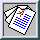Physics of Fluids A -- October 1989 -- Volume 1, Issue 10, pp. 1698-1700

### Turbulence spectrum of strongly conductive temperature field in a rapidly stirred fluid

J. R. Chasnov, V. M. Canuto and R. S. Rogallo
NASA Goddard Institute for Space Studies, New York, USA

In an earlier paper [Phys. Fluids 31, 2065 (1988)], a numerical simulation of a passive scalar field convected by a frozen velocity field, i.e., a velocity field with an infinite correlation time, was performed. In this paper, a simulation of a passive scalar field convected by a velocity field which is rapidly stirred at all scales of motion, i.e., a velocity field with near zero correlation time, is performed. For an energy spectrum of the form k-5/3, the temperature spectrum is found to obey k-11/3 when conductive effects are dominant. A theoretical model is proposed which obtains the above result by representing the transfer of scalar variance by an eddy conductivity, whose correlation time is limited by the correlation time of the velocity field.

Full Text: [PDF (320 KB)]